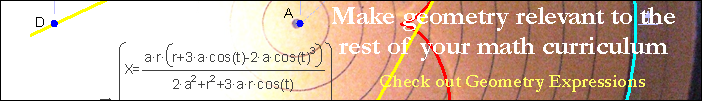CircleMinor ArcA minor arc is an arc of a circle with a measure of less than or equal to 180 degrees.Major ArcA major arc is an arc of a circle with a measure of greater than or equal to 180 degreesArbelosThe arbelos or "cobbler's knife" is a figure made up from 3 semi circles.Arc Length, DegreesArc length is a fraction of the circumference.Intersecting ChordsThe measure of the angle made by intersecting chords is equal to the average of the measures of the subtended arcs.Intersecting SecantsIf secants intersect outside the circle, the angle between the secants is equal to the difference of the measures of the subtended arcs divided by two.Circular Functions: Sine and CosineAny point on the unit circle has the coordinates (cos(θ), sin(θ)).